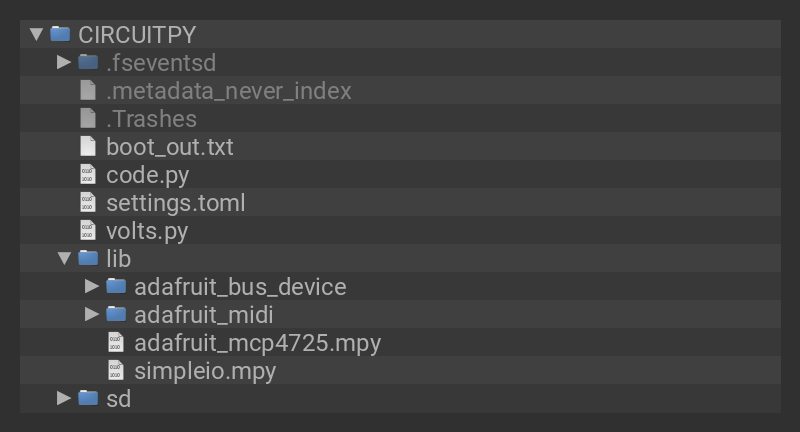Once you've finished setting up your QT Py RP2040 with CircuitPython, you can access the code and necessary libraries by downloading the Project Bundle.

```# SPDX-FileCopyrightText: 2022 Liz Clark for Adafruit Industries

import board
import simpleio
import usb_midi
from digitalio import DigitalInOut, Direction
from volts import volts

#  midi channel setup
midi_in_channel = 1
midi_out_channel = 1

#  USB midi setup
midi_in=usb_midi.ports, in_channel=0, midi_out=usb_midi.ports, out_channel=0
)

# gate output pin
gate = DigitalInOut(board.A1)
gate.direction = Direction.OUTPUT

#  i2c setup
i2c = board.I2C()  # uses board.SCL and board.SDA
# i2c = board.STEMMA_I2C()  # For using the built-in STEMMA QT connector on a microcontroller
#  dac setup over i2c

#  dac raw value (12 bit)
dac.raw_value = 4095

#  array for midi note numbers
midi_notes = []
#  array for 12 bit 1v/oct values
pitches = []

#  function to map 1v/oct voltages to 12 bit values
#  these values are added to the pitches[] array
def map_volts(n, volt, vref, bits):
n = simpleio.map_range(volt, 0, vref, 0, bits)
pitches.append(n)

#  brings values from volts.py into individual arrays
for v in volts:
#  map_volts function to map 1v/oct values to 12 bit
#  and append to pitches[]
map_volts(v['label'], v['1vOct'], 5, 4095)
#  append midi note numbers to midi_notes[] array
midi_notes.append(v['midi'])

while True:
#  if a midi msg comes in...
if msg is not None:
#  if it's noteoff...
if isinstance(msg, NoteOff):
#  send 0 volts on dac
dac.raw_value = 0
#  turn off gate pin
gate.value = False
#  if it's noteon...
if isinstance(msg, NoteOn):
#  compare incoming note number to midi_notes[]
z = midi_notes.index(msg.note)
#  limit note range to defined notes in volts.py
if msg.note < 36:
msg.note = 36
if msg.note > 96:
msg.note = 96
#  send corresponding 1v/oct value
dac.raw_value = int(pitches[z])
#  turn on gate pin
gate.value = True
```

## Upload the Code and Libraries to the QT Py RP2040

After downloading the Project Bundle, plug your QT Py RP2040 into the computer's USB port with a known good USB data+power cable. You should see a new flash drive appear in the computer's File Explorer or Finder (depending on your operating system) called CIRCUITPY. Unzip the folder and copy the following items to the QT Py RP2040's CIRCUITPY drive.

• lib folder
• volts.py
• code.py

Your QT Py RP2040 CIRCUITPY drive should look like this after copying the lib folder, volts.py file and the code.py file.## volts.pyFile

The volts.py file is a helper file that contains a dictionary (`volts[]`) with the phenetic note name, the MIDI note number and the 1V/oct voltage. The range of notes are C2 to C7, or 0V to 5V.

```volts = [
{'label':"C-2",'midi':36,'1vOct':0.000},
{'label':"C♯2",'midi':37,'1vOct':0.083},
{'label':"D-2",'midi':38,'1vOct':0.167},
...
{'label':"B-6",'midi':95,'1vOct':4.917},
{'label':"C-7",'midi':96,'1vOct':5.000},
]```

## How the CircuitPython Code Works

First, USB MIDI, the digital output for the gate signal, I2C and the DAC are setup. Note that `board.I2C()` is being used rather than `STEMMA_I2C()`.

```#  midi channel setup
midi_in_channel = 1
midi_out_channel = 1

#  USB midi setup
midi_in=usb_midi.ports, in_channel=0, midi_out=usb_midi.ports, out_channel=0
)

# gate output pin
gate = DigitalInOut(board.A1)
gate.direction = Direction.OUTPUT

#  i2c setup
i2c = board.I2C()
#  dac setup over i2c

#  dac raw value (12 bit)
dac.raw_value = 4095```

## Mapping Voltages

Two arrays are created: `midi_notes[]` and `pitches[]`. `midi_notes[]` will hold the MIDI note numbers as defined in volts.py. `pitches[]` will hold the 12-bit DAC values that correspond with the 1V/oct values in volts.py.

```#  array for midi note numbers
midi_notes = []
#  array for 12 bit 1v/oct values
pitches = []```

The `map_volts()` function, uses simpleio's `map_range()` function to map the 1V/oct values from volts.py to 12-bit values for the MCP4725 DAC. These 12-bit values are then added to the `pitches[]` array.

```#  function to map 1v/oct voltages to 12 bit values
#  these values are added to the pitches[] array
def map_volts(n, volt, vref, bits):
n = simpleio.map_range(volt, 0, vref, 0, bits)
pitches.append(n)```

A `for` statement iterates through the dictionary in volts.py and uses `map_volts()` to map the 1V/oct values to 12-bit values. Then, the MIDI note numbers in volts.py are added to the `midi_notes[]` array.

The indexes of each array correspond with each other for the same note and will be used in the loop. For example, `midi_notes` equals MIDI note `36` and `pitches` equals `0`, which are two different ways of saying note C2.

```#  brings values from volts.py into individual arrays
for v in volts:
#  map_volts function to map 1v/oct values to 12 bit
#  and append to pitches[]
map_volts(v['label'], v['1vOct'], 5, 4095)
#  append midi note numbers to midi_notes[] array
midi_notes.append(v['midi'])```

## The Loop

The loop begins by listening to incoming MIDI messages. In this instance of the code, the specific messages being listened for are `NoteOn` and `NoteOff` messages.

If a `NoteOn` message is received, the message's note number is checked against the `midi_notes` array and the matching index is defined as `z`. The DAC's value is set to `pitches[z]`, sending the corresponding 1V/oct voltage as a 12-bit value. `gate`'s value is set to `True`.

If a `NoteOff` message is received, the DAC's value is set to `0` and `gate`'s value is set to `False`

```while True:
#  if a midi msg comes in...
if msg is not None:
#  if it's noteoff...
if isinstance(msg, NoteOff):
#  send 0 volts on dac
dac.raw_value = 0
#  turn off gate pin
gate.value = False
#  if it's noteon...
if isinstance(msg, NoteOn):
#  compare incoming note number to midi_notes[]
z = midi_notes.index(msg.note)
#  limit note range to defined notes in volts.py
if msg.note < 36:
msg.note = 36
if msg.note > 96:
msg.note = 96
#  send corresponding 1v/oct value
dac.raw_value = int(pitches[z])
#  turn on gate pin
gate.value = True```

This guide was first published on Aug 02, 2022. It was last updated on Jul 29, 2022.

This page (Code the MIDI to CV Skull) was last updated on Sep 30, 2023.